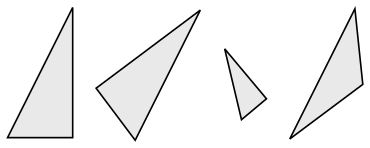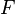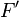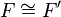# Congruence facts for kids

Kids Encyclopedia FactsAn example of congruence. The two triangles on the left are congruent, while the third is similar to them. The last triangle is neither similar nor congruent to any of the others. Note that congruence permits alteration of some properties, such as location and orientation, but leaves others unchanged, like distance and angles. The unchanged properties are called invariants.

In geometry, two figures or objects$F$ and$F'$ are congruent (written as$F \cong F'$) if they have the same shape and size, or if one has the same shape and size as the mirror image of the other.

More formally, two sets of points are called congruent, if and only if one can be transformed into the other by isometry. For isometry, rigid motions are used.

This means that two geometrical figures are congruent if one object can be repositioned, rotated or reflected—but not resized—so that it coincides exactly with the other object. if one can be moved or rotated so that it fits exactly where the other one is, then the two figures are congruent. If one of the object has to change its size, then the two objects are not congruent: they are just called similar.

As an example, two distinct plane figures on a piece of paper are congruent if we can cut them out and then match them up completely (turning the paper over here is permitted).

Congruent polygons are polygons that if you fold a regular polygon in half that is a congruent polygon.

## Examples

• All squares that have the same length of their sides are congruent.
• All equilateral triangles that have the same length of their sides are congruent.

## Tests for congruency

• Two angles and the side between them are the same on two triangles (ASA congruence)
• Two angles and a side not between them are the same on both triangles (AAS congruence)
• All three sides of both triangles are the same (SSS congruence)
• Two sides and the angle between them makes two triangles congruent (SAS congruence)

## How to obtain new congruent shapes

The following are a few rules to make new shapes congruent to the original one:

• If we shift a geomentrical shape in the plane, then we get a shape which is congruent to the original one.
• If we rotate instead of shifting, then we also get a shape congruent to the original one.
• Even if we take a mirror image of the original shape, then we still get a congruent shape.
• If we combine the three activities one after the other, then we still get congruent shapes.
• There are no more congruent shapes. More specifically, if a shape is congruent to the original one, then it can be reached by the three activities described above.

The relationship, that a shape is congruent to another shape, has three famous properties:

• If we leave the original shape alone at its original place, then it is congruent to itself. This property is called reflexivity.
For example, if the shift above is not a proper shift, but only a shift making a motion of length zero. Or, similarly, if the rotation above is not a proper rotation, but only a rotation of angle zero.
• If a shape is congruent to another shape, then this other shape is also congruent to the original one. This behaviour, this property is called symmetry.
For example, if we shift back, or rotate back, or mirror back the new shape to the original one, then the original shape is congruent to the new one.
• If a shape C is congruent to a shape B, and the shape B is congruent to the original shape A, then the shape C is also congruent to the original shape A. This property is called transitivity.
For example, if we apply first a shift, and then a rotation, then the resulting new shape is still congruent to the original one.

The famous three properties, reflexivity, symmetry and transitivity, together make the notion of equivalence. Hence, the property congruence is one sort of equivalence relation between shapes of a plane.

## Related pagesCongruence Facts for Kids. Kiddle Encyclopedia.